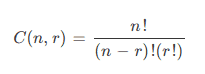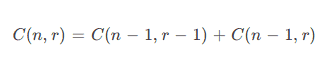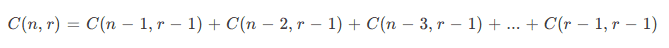### Get started with Spring 5 and Spring Boot 2, through the Learn Spring course:

>> CHECK OUT THE COURSE

## 1. Introduction

In this tutorial, we'll discuss the solution to the k-combinations problem in Java.

First, we'll discuss and implement both recursive and iterative algorithms to generate all combinations of a given size. Then we'll review solutions using common Java libraries.

## 2. Combinations Overview

Simply put, a combination is a subset of elements from a given set.

Unlike permutations, the order in which we choose the individual elements doesn't matter. Instead, we only care whether a particular element is in the selection.

For example, in a card game, we have to deal 5 cards out of the pack consisting of 52 cards. We have no interest in the order in which the 5 cards were selected. Rather, we only care which cards are present in the hand.

Some problems require us to evaluate all possible combinations. In order to do this, we enumerate the various combinations.

The number of distinct ways to choose “r” elements from the set of “n” elements can be expressed mathematically with the following formula:Therefore, the number of ways to choose elements can grow exponentially in the worst case. Hence, for large populations, it may not be possible to enumerate the different selections.

In such cases, we may randomly select a few representative selections. The process is called sampling.

Next, we'll review the various algorithms to list combinations.

## 3. Recursive Algorithms to Generate Combinations

Recursive algorithms usually work by partitioning a problem into similar smaller problems. This process continues until we reach the terminating condition, which is also the base case. Then we solve the base case directly.

We'll discuss two ways to subdivide the task of choosing elements from a set. The first approach divides the problem in terms of the elements in the set. The second approach divides the problem by tracking the selected elements only.

### 3.1. Partitioning by Elements in the Entire Set

Let's divide the task of selecting “r” elements from “n” items by inspecting the items one by one. For each item in the set, we can either include it in the selection or exclude it.

If we include the first item, then we need to choose “r – 1″ elements from the remaining “n – 1″ items. On the other hand, if we discard the first item, then we need to select “r” elements out of the remaining “n – 1″ items.

This can be mathematically expressed as:Now, let's look into the recursive implementation of this approach:

``````private void helper(List<int[]> combinations, int data[], int start, int end, int index) {
if (index == data.length) {
int[] combination = data.clone();
} else if (start <= end) {
data[index] = start;
helper(combinations, data, start + 1, end, index + 1);
helper(combinations, data, start + 1, end, index);
}
}``````

The helper method makes two recursive calls to itself. The first call includes the current element. The second call discards the current element.

Next, let's write the combination generator using this helper method:

``````public List<int[]> generate(int n, int r) {
List<int[]> combinations = new ArrayList<>();
helper(combinations, new int[r], 0, n-1, 0);
return combinations;
}``````

In the above code, the generate method sets up the first call to the helper method and passes the appropriate parameters.

Next, let's call this method to generate combinations:

``````List<int[]> combinations = generate(N, R);
for (int[] combination : combinations) {
System.out.println(Arrays.toString(combination));
}
System.out.printf("generated %d combinations of %d items from %d ", combinations.size(), R, N);``````

On executing the program, we get the following output:

``````[0, 1]
[0, 2]
[0, 3]
[0, 4]
[1, 2]
[1, 3]
[1, 4]
[2, 3]
[2, 4]
[3, 4]
generated 10 combinations of 2 items from 5``````

Finally, let's write the test case:

``````@Test
public void givenSetAndSelectionSize_whenCalculatedUsingSetRecursiveAlgorithm_thenExpectedCount() {
SetRecursiveCombinationGenerator generator = new SetRecursiveCombinationGenerator();
List<int[]> selection = generator.generate(N, R);
assertEquals(nCr, selection.size());
}``````

It is easy to observe that the stack size required is the number of elements in the set. When the number of elements in the set is large, say, greater than the maximum call stack depth, we'll overflow the stack and get a StackOverflowError.

Therefore, this approach doesn't work if the input set is large.

### 3.2. Partitioning by Elements in the Combination

Instead of tracking the elements in the input set, we'll divide the task by tracking the items in the selection.

First, let's order the items in the input set using indices “1” to “n”. Now, we can choose the first item from the first “n-r+1″ items.

Let's assume that we chose the kth item. Then, we need to choose “r – 1″ items from the remaining “n – k” items indexed “k + 1″ to “n”.

We express this process mathematically as:Next, let's write the recursive method to implement this approach:

``````private void helper(List<int[]> combinations, int data[], int start, int end, int index) {
if (index == data.length) {
int[] combination = data.clone();
} else {
int max = Math.min(end, end + 1 - data.length + index);
for (int i = start; i <= max; i++) {
data[index] = i;
helper(combinations, data, i + 1, end, index + 1);
}
}
}``````

In the above code, the for loop chooses the next item, Then, it calls the helper() method recursively to choose the remaining items. We stop when the required number of items have been selected.

Next, let's use the helper method to generate selections:

``````public List<int[]> generate(int n, int r) {
List<int[]> combinations = new ArrayList<>();
helper(combinations, new int[r], 0, n - 1, 0);
return combinations;
}``````

Finally, let's write a test case:

``````@Test
public void givenSetAndSelectionSize_whenCalculatedUsingSelectionRecursiveAlgorithm_thenExpectedCount() {
SelectionRecursiveCombinationGenerator generator = new SelectionRecursiveCombinationGenerator();
List<int[]> selection = generator.generate(N, R);
assertEquals(nCr, selection.size());
}``````

The call stack size used by this approach is the same as the number of elements in the selection. Therefore, this approach can work for large inputs so long as the number of elements to be selected is less than the maximum call stack depth.

If the number of elements to be chosen is also large, this method won't work.

## 4. Iterative Algorithm

In the iterative approach, we start with an initial combination. Then, we keep generating the next combination from the current one until we have generated all combinations.

Let's generate the combinations in lexicographic order. We start with the lowest lexicographic combination.

In order to get the next combination from the current one, we find the rightmost location in the current combination that can be incremented. Then, we increment the location and generate the lowest possible lexicographic combination to the right of that location.

Let's write the code which follows this approach:

``````public List<int[]> generate(int n, int r) {
List<int[]> combinations = new ArrayList<>();
int[] combination = new int[r];

// initialize with lowest lexicographic combination
for (int i = 0; i < r; i++) {
combination[i] = i;
}

while (combination[r - 1] < n) {

// generate next combination in lexicographic order
int t = r - 1;
while (t != 0 && combination[t] == n - r + t) {
t--;
}
combination[t]++;
for (int i = t + 1; i < r; i++) {
combination[i] = combination[i - 1] + 1;
}
}

return combinations;
}``````

Next, let's write the test case:

``````@Test
public void givenSetAndSelectionSize_whenCalculatedUsingIterativeAlgorithm_thenExpectedCount() {
IterativeCombinationGenerator generator = new IterativeCombinationGenerator();
List<int[]> selection = generator.generate(N, R);
assertEquals(nCr, selection.size());
}``````

Now, let us use some Java libraries to solve the problem.

## 5. Java Libraries Implementing Combinations

As far as possible, we should reuse existing library implementations instead of rolling out our own. In this section, we'll explore the following Java libraries that implement combinations:

• Apache Commons
• Guava
• CombinatoricsLib

### 5.1. Apache Commons

The CombinatoricsUtils class from Apache Commons provides many combination utility functions. In particular, the combinationsIterator method returns an iterator that will generate combinations in lexicographic order.

First, let's add the Maven dependency commons-math3 to the project:

``````<dependency>
<groupId>org.apache.commons</groupId>
<artifactId>commons-math3</artifactId>
<version>3.6.1</version>
</dependency>``````

Next, let's use the combinationsIterator method to print the combinations:

``````public static void generate(int n, int r) {
Iterator<int[]> iterator = CombinatoricsUtils.combinationsIterator(n, r);
while (iterator.hasNext()) {
final int[] combination = iterator.next();
System.out.println(Arrays.toString(combination));
}
}``````

The Sets class from Guava library provides utility methods for set-related operations. The combinations method returns all subsets of a given size.

First, let's add the maven dependency for the Guava library to the project:

``````<dependency>
<artifactId>guava</artifactId>
<version>31.0.1-jre</version>
</dependency>``````

Next, let's use the combinations method to generate combinations:

``Set<Set<Integer>> combinations = Sets.combinations(ImmutableSet.of(0, 1, 2, 3, 4, 5), 3);``

Here, we are using the ImmutableSet.of method to create a set from the given numbers.

### 5.3. CombinatoricsLib

CombinatoricsLib is a small and simple Java library for permutations, combinations, subsets, integer partitions, and cartesian product.

To use it in the project, let's add the combinatoricslib3 Maven dependency:

``````<dependency>
<groupId>com.github.dpaukov</groupId>
<artifactId>combinatoricslib3</artifactId>
<version>3.3.0</version>
</dependency>``````

Next, let's use the library to print the combinations:

``````Generator.combination(0, 1, 2, 3, 4, 5)
.simple(3)
.stream()
.forEach(System.out::println);``````

This produces the following output on execution:

``````[0, 1, 2]
[0, 1, 3]
[0, 1, 4]
[0, 1, 5]
[0, 2, 3]
[0, 2, 4]
[0, 2, 5]
[0, 3, 4]
[0, 3, 5]
[0, 4, 5]
[1, 2, 3]
[1, 2, 4]
[1, 2, 5]
[1, 3, 4]
[1, 3, 5]
[1, 4, 5]
[2, 3, 4]
[2, 3, 5]
[2, 4, 5]
[3, 4, 5]``````

More examples are available at combinatoricslib3-example.